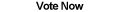Home Articles Components Code Snippets Links# C# Calculate Distance Formula

## Distance Formula

There is not automate to calculate distance formula equations automatically in C#. Yet it is a simple enough calculation that we can write a simple accurate C# function...

The distance formula lets programmers calculate the distance between to points in the coordinate system. While there is a distance formula for 3D and 2D systems, in this article we'll focus on the 2D distance formula.

## Calculating

To calculate the distance between two points, we need to make use of the pythagorean theorem. The theorem states that the two short sides of a triangle, squared and added, will equal the square of the longest side.

So by subtracting the X values of two points, you can get one side of the triangle. Subtract the Y values to get the other side. Then use the Pythagorean Theorem to calculate the last side of the triangle, which will be the distance between to points.

So in essence, to calculate distance formula equations, you just need careful math.

## C# Distance Function

You can see the actual formula in the source code that is downloadable at the bottom of the page. You will notice that the formula uses PointF objects and the double primitive type. This is to make calculate distance formula equations more accurate. However feel free to accomodate it to integers.

Back to C# Article List